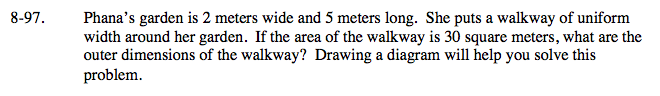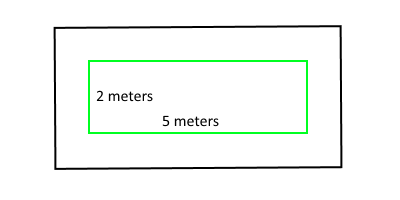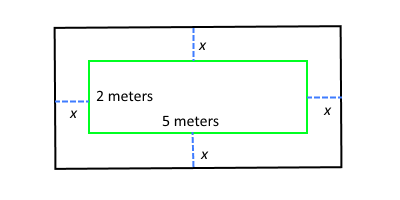### Home > A2C > Chapter 8 > Lesson 8.1.6 > Problem8-97

8-97.Draw a diagram.

Find the total area of the outside rectangle by adding
the area of the inside rectangle to the area of the walkway.

30 + 10 = 40 sq m

If x meters of walkway was added to each side, write an
expression for each side of the outside rectangle.

length = 5 + 2x
width = 2 + 2xNow write an equation of the form (length)(width) = area and solve for x.
Make sure you remember to answer the original question.